#0
0
01. 云栖社区>
2. 博客>
3. 正文

## 如何用Python智能批量压缩图片？# 痛点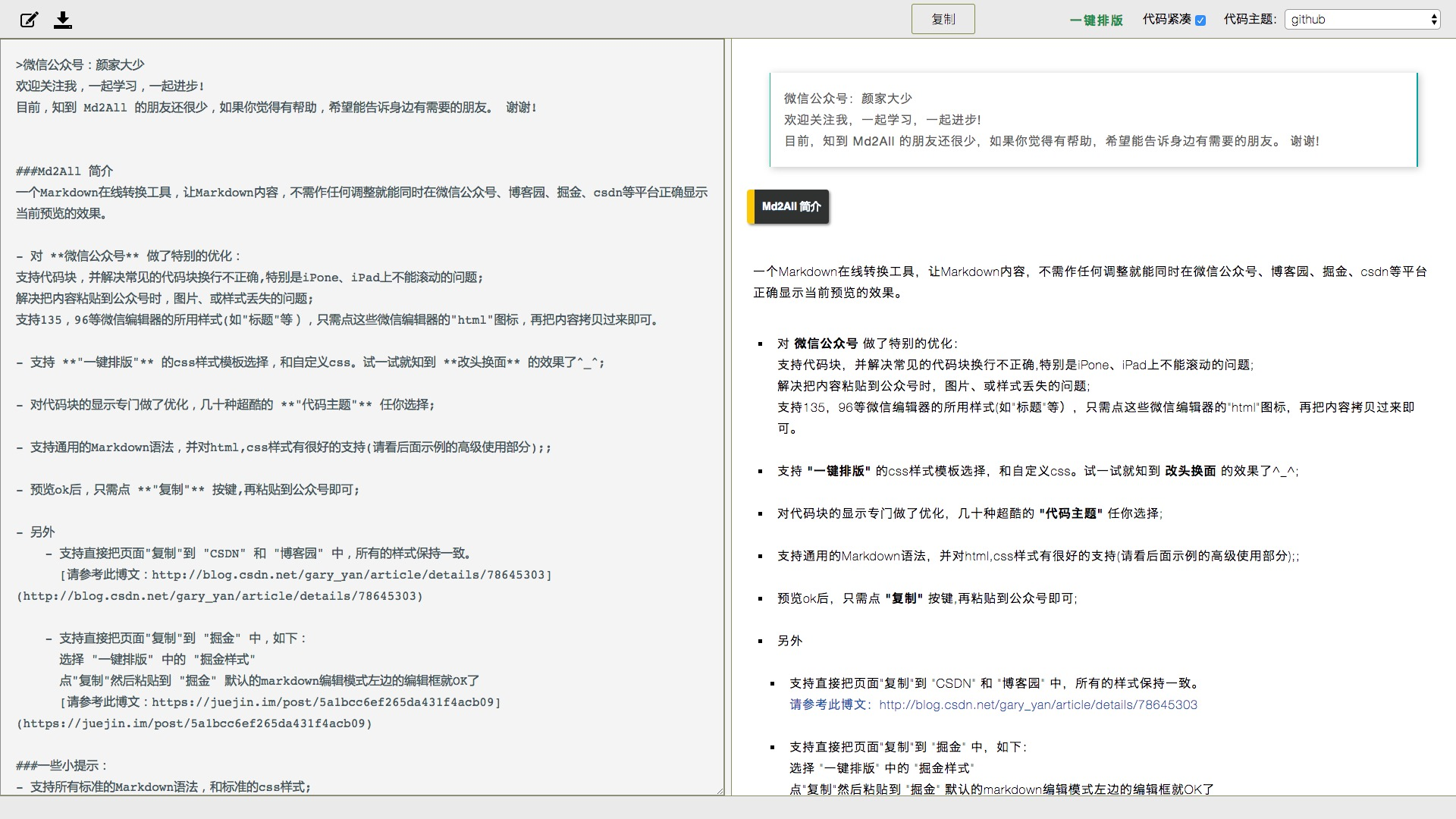# 数据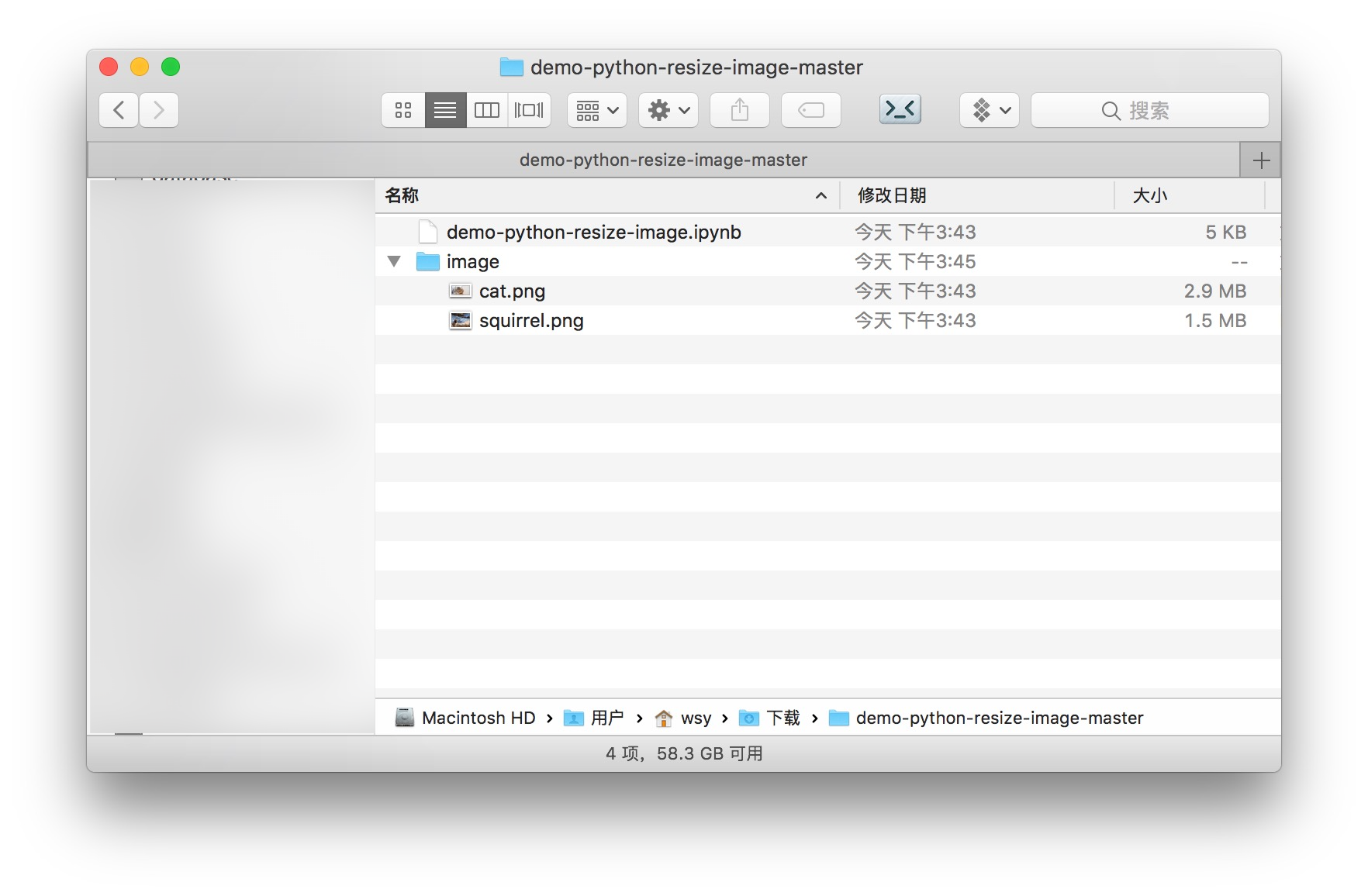# 环境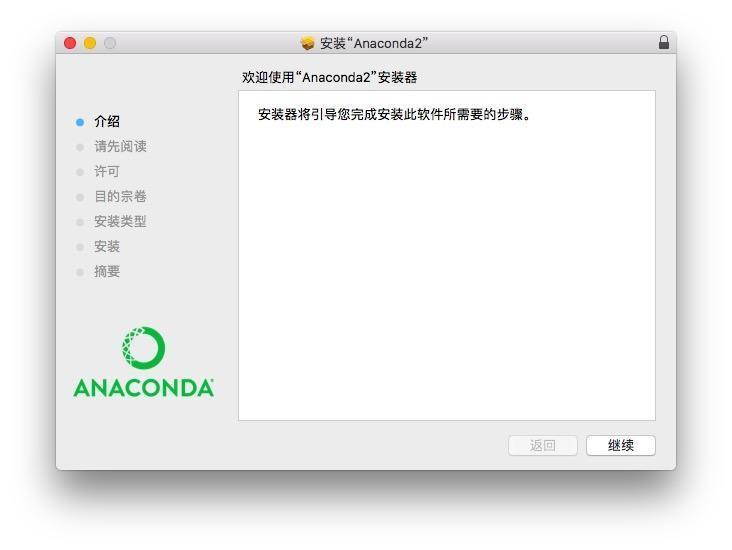``````pip install -U PIL
pip install -U glob
``````

``````jupyter notebook
``````# 代码

``````from glob import glob
from PIL import Image
import os
``````

``````source_dir = 'image'
``````

``````target_dir = 'output'
``````

``````filenames = glob('{}/*'.format(source_dir))
``````

``````print(filenames)
``````
``````['image/squirrel.png', 'image/cat.png']
``````

``````for filename in filenames:
with Image.open(filename) as im:
width, height = im.size
print(filename, width, height, os.path.getsize(filename))
``````

``````('image/squirrel.png', 1024, 768, 1466487)
('image/cat.png', 2067, 1163, 2851538)
``````

``````2*1024*1024
``````

``````2097152
``````

``````threshold = 2*1024*1024
``````

``````for filename in filenames:
filesize = os.path.getsize(filename)
if filesize >= threshold:
print(filename)
``````

``````image/cat.png
``````

``````if not os.path.exists(target_dir):
os.makedirs(target_dir)
``````

``````for filename in filenames:
filesize = os.path.getsize(filename)
if filesize >= threshold:
print(filename)
with Image.open(filename) as im:
width, height = im.size
new_width = 1024
new_height = int(new_width * height * 1.0 / width)
``````

``````image/cat.png
``````

``````for filename in filenames:
filesize = os.path.getsize(filename)
if filesize >= threshold:
print(filename)
with Image.open(filename) as im:
width, height = im.size
new_width = 1024
new_height = int(new_width * height * 1.0 / width)
resized_im = im.resize((new_width, new_height))
output_filename = filename.replace(source_dir, target_dir)
resized_im.save(output_filename)
``````

``````image/cat.png
``````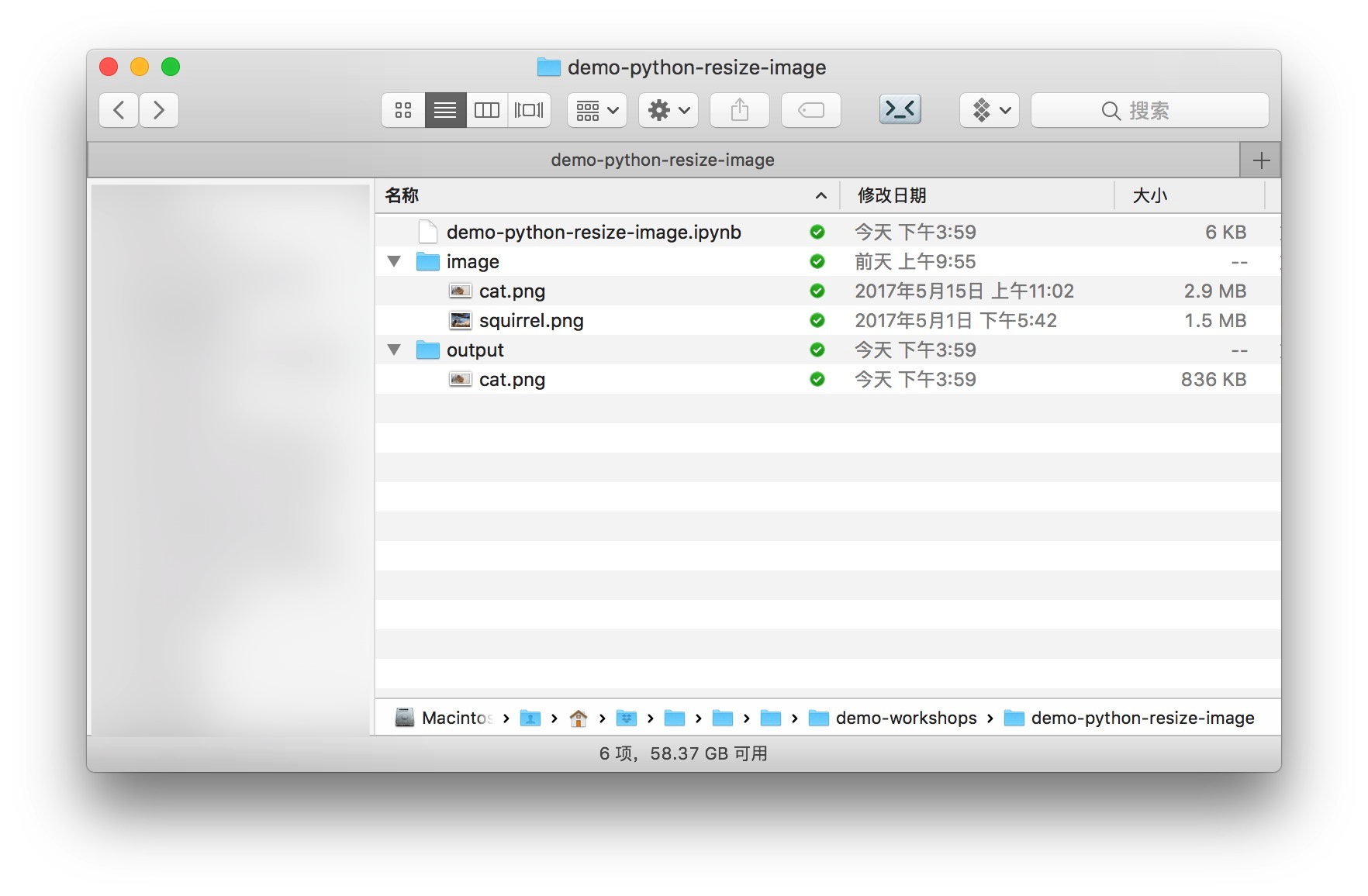# 整合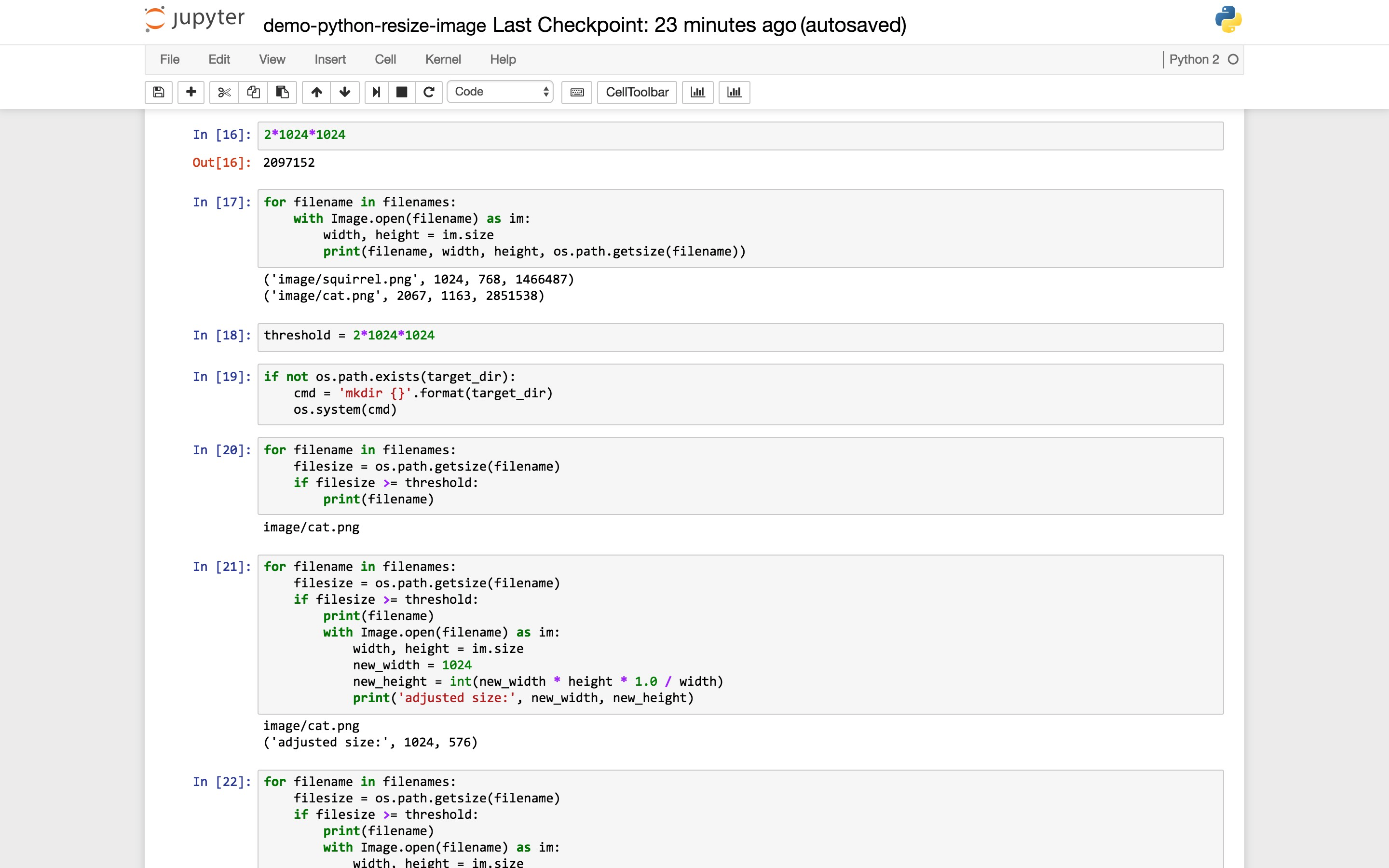``````def resize_images(source_dir, target_dir, threshold):
filenames = glob('{}/*'.format(source_dir))
if not os.path.exists(target_dir):
os.makedirs(target_dir)
for filename in filenames:
filesize = os.path.getsize(filename)
if filesize >= threshold:
print(filename)
with Image.open(filename) as im:
width, height = im.size
new_width = 1024
new_height = int(new_width * height * 1.0 / width)
resized_im = im.resize((new_width, new_height))
output_filename = filename.replace(source_dir, target_dir)
resized_im.save(output_filename)
``````

• `source_dir`：图片源目录
• `target_dir`：压缩图片输出目录
• `threshold`：阈值

``````import math
``````

``````def resize_images(source_dir, target_dir, threshold):
filenames = glob('{}/*'.format(source_dir))
if not os.path.exists(target_dir):
os.makedirs(target_dir)
for filename in filenames:
filesize = os.path.getsize(filename)
if filesize >= threshold:
print(filename)
with Image.open(filename) as im:
width, height = im.size
if width >= height:
new_width = int(math.sqrt(threshold/2))
new_height = int(new_width * height * 1.0 / width)
else:
new_height = int(math.sqrt(threshold/2))
new_width = int(new_height * width * 1.0 / height)
resized_im = im.resize((new_width, new_height))
output_filename = filename.replace(source_dir, target_dir)
resized_im.save(output_filename)
``````

``````resize_images(source_dir, target_dir, threshold)
``````

``````image/cat.png
``````# 小结

• 如何利用glob软件包遍历指定目录，获得符合条件的全部文件路径列表；
• 如何用PIL图像处理工具读取图像文件，检查宽度、高度，重新设定图像大小，并且存储新生成的图像；
• 如何用os函数库检查文件或目录是否存在，创建目录，以及获取文件尺寸。

+ 关注